# Speed and Velocity

(This article was translated from Japanese to English using DeepL.)

The theme of this lecture is “Let’s correctly distinguish between similar terms.”

In this lecture, we will learn about the difference between speed and velocity, which is the title of the lecture.

## Difference in meaning between Speed and Velocity

Let’s quickly look at the difference between these two terms. See the following example.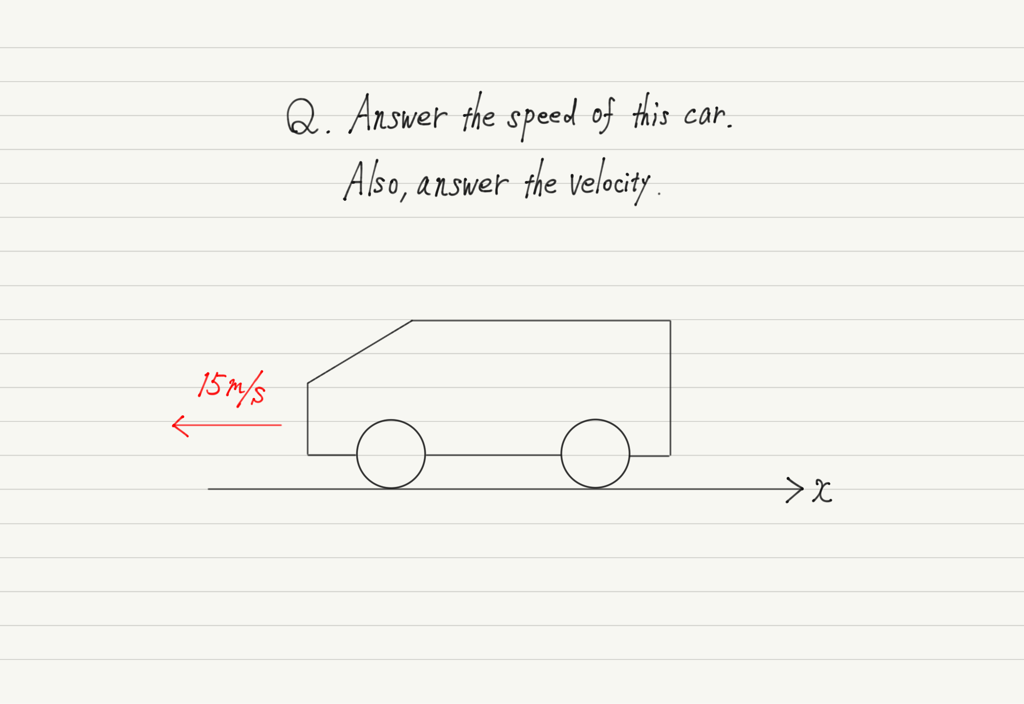What would be the answer to this question?
Is the speed or the velocity, both 15 m/s?

That is not true. The correct answer is below.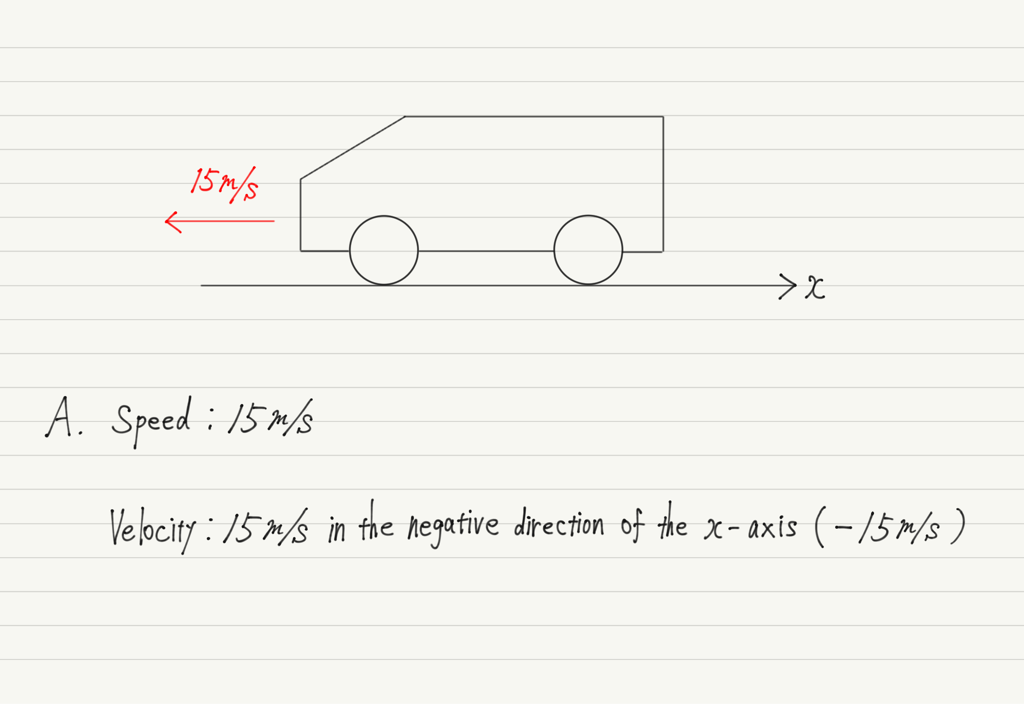For speed, the answer is simply the numerical value “15 m/s,” whereas for velocity, the direction must also be added.
The direction of motion is very important information in the study of motion, because even if the speed is the same, different directions will lead to different arrival points.

By the way, the fact that there is a direction means that velocity can be represented by an arrow.
Promise me that “the direction of the arrow indicates the direction of velocity, and the longer the arrow, the faster.”

Velocity and speed are used with the same meaning in everyday life, but in physics they have different meanings.
When reading textbooks and reference books and solving problems, be sure to pay attention to which one is being used!

## 【Example】 Resultant velocity

The direction of motion is also important in the calculation. As one such example, let’s compute the resultant velocity!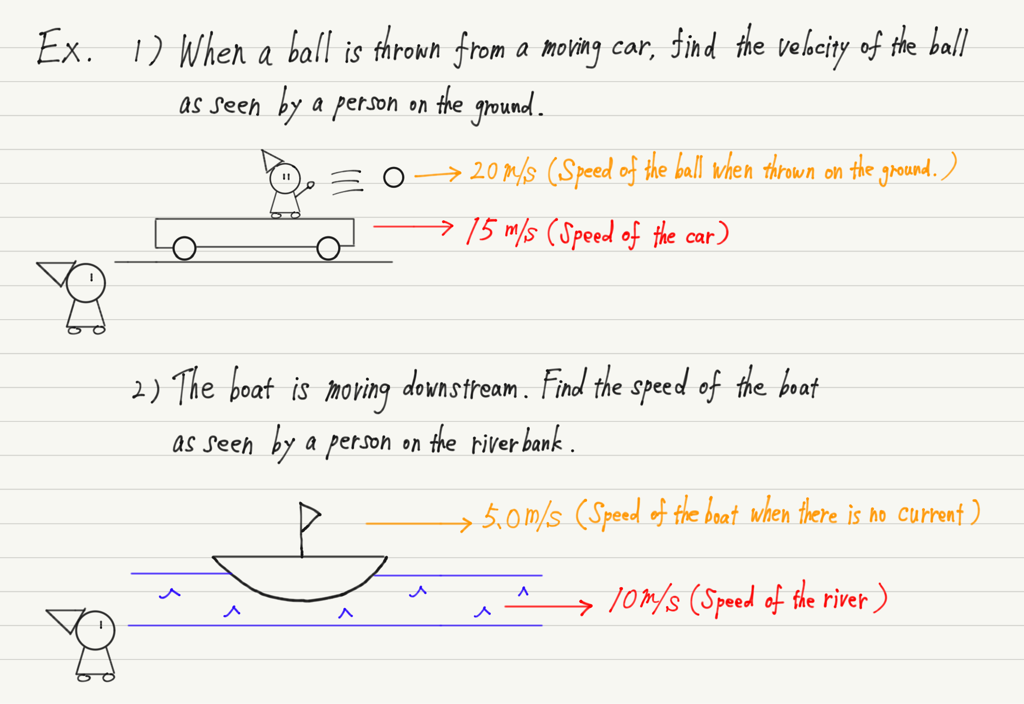The idea is very simple.

When object A is in motion and object B is in motion above it, the velocity of B from the outside is the velocity of B plus the velocity of A (= resultant velocity).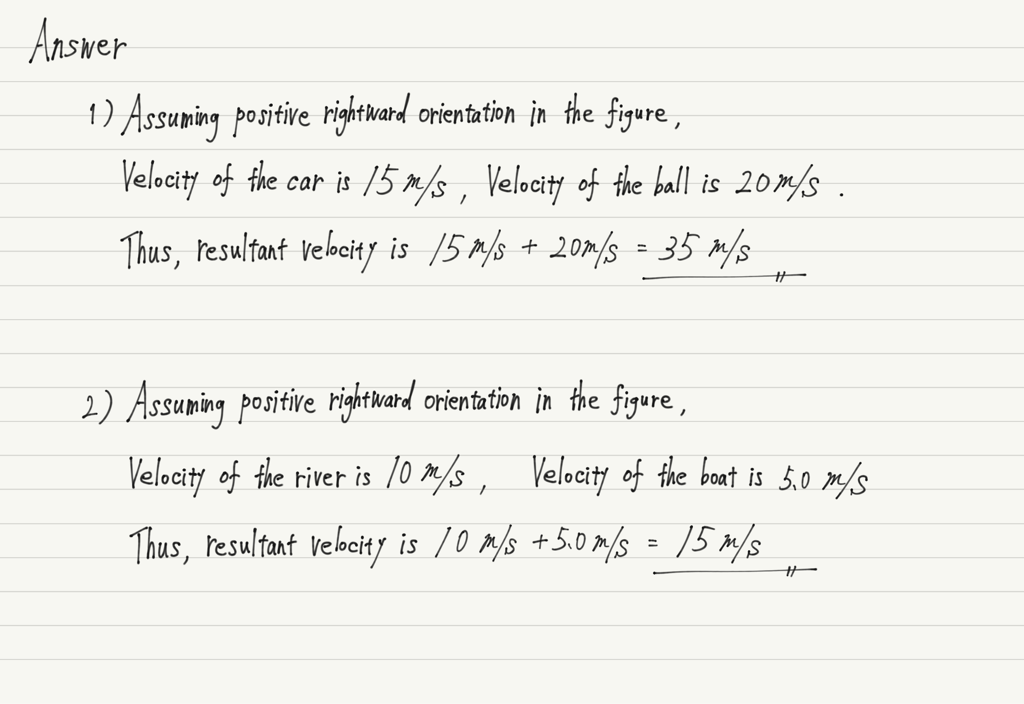Now, let’s change the problem set up a bit.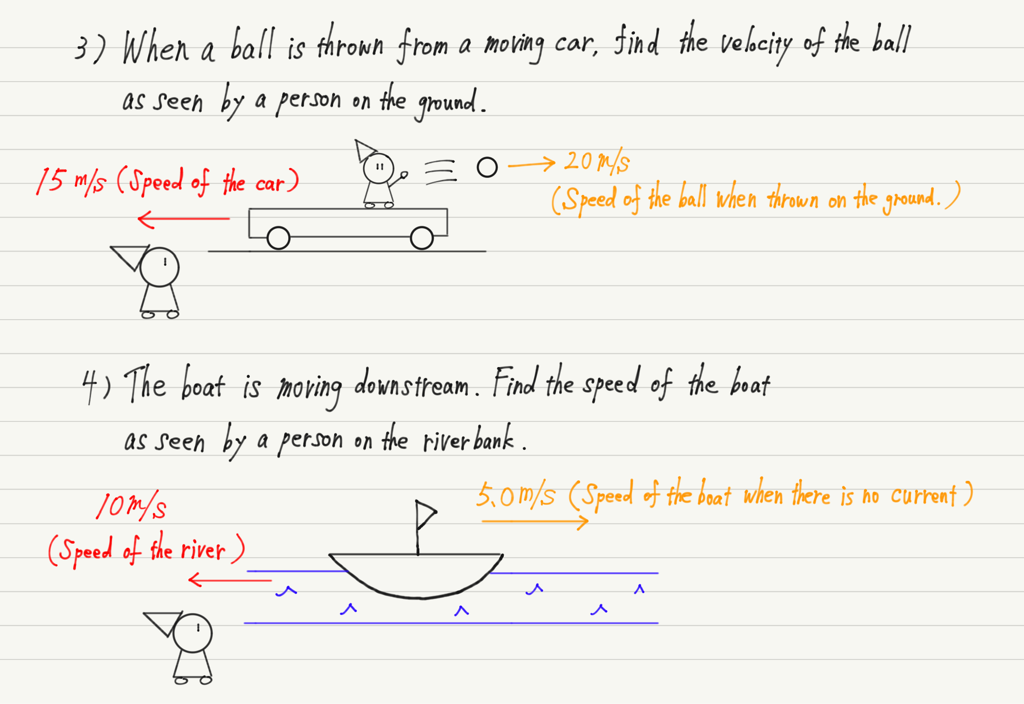In the previous example, we could just add the numerical values, but not this time, because A and B are moving in opposite directions!
Let’s represent the opposite direction with a minus sign and then do the calculation.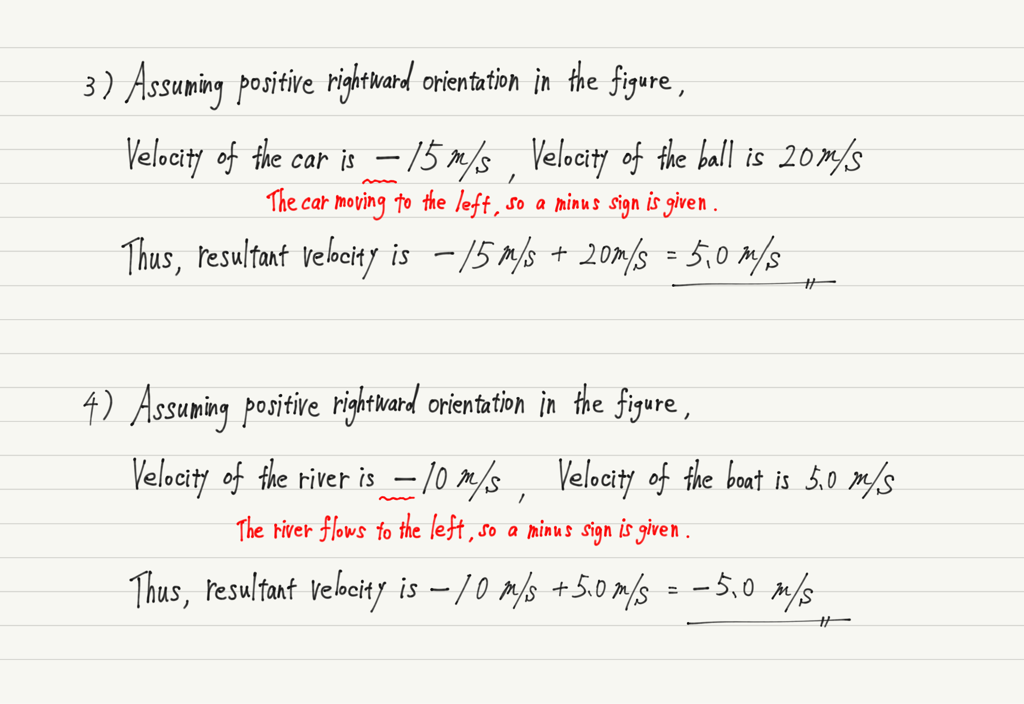The answers to 3) and 4) would be totally different without the minus. You can see that orientation plays an important role in our investigation of motion!

## Summary of this lecture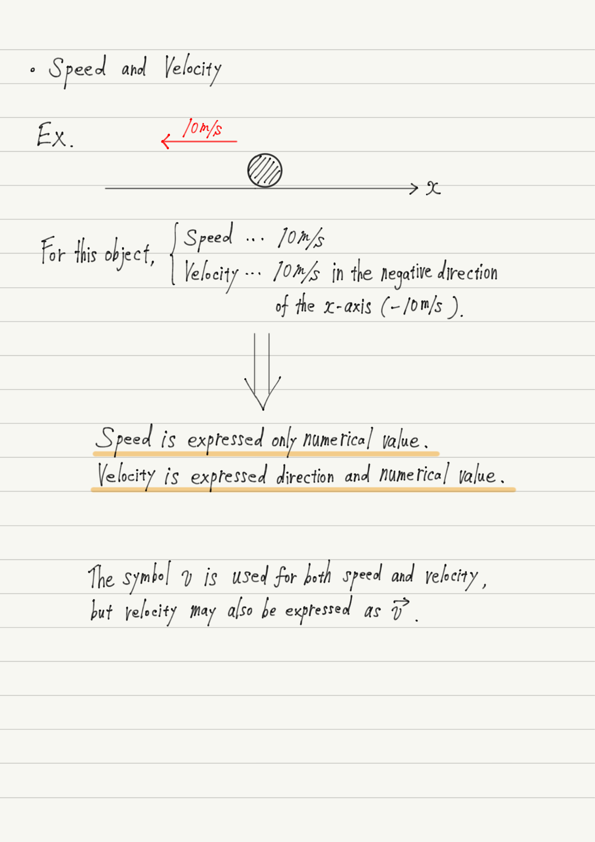## Next Time

The next lecture will continue to cover similar terms.

https://www.yukimura-physics.com/entry/dyn-f04

error: Content is protected !!